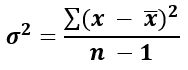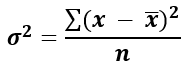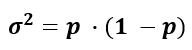# Variance Calculator

Use this calculator to easily calculate the variance of a sample, or to estimate the population variance based on a random sample from it. Variance of binomial data. The calculator also outputs the standard deviation, mean, range, count, and SEM.

Share calculator:

Embed this tool:
get code

## What is variance?

Variance is a term in statistics and probability theory used to quantify the amount of dispersion in a numerical data set, that is - how far from the normal (average) are the data points of interest. Variance may be written as Var() or by the small Greek letter notation σ2 when referencing a population estimate based on a sample and the Latin s2 when referencing sample variance which is directly calculated.

A low variance σ2 means that the data points are clustered more closely to the sample mean while a high variance indicates that the set of data is spread over a wider range of values.

## How to calculate variance

To calculate variance, take the arithmetic mean of the differences between each data point and the dataset mean. The differences are squared so that larger distances from the mean are threated exponentially more severely, as well as treating discrepancies in both the positive and negative direction equally.

If the standard deviation is known, the variance can be calculated by squaring it (raising it to the power of two). Typically the standard deviation is the preferred measure of dispersion since it is expressed in the original measurement units, unlike the variance. Our variance calculator will also output the standard deviation.

For continuous variables the whole raw dataset is required while for binomial data like proportions the variance can be calculated analytically using two summary statistics: the number of observations (sample size) and the rate of events of interest (which is also a mean).

## Variance formulas

There are two main variance formulas. Which one is appropriate depends on whether one wants to estimate the variance based on a sample from a population or to find the variance of a whole population that can be directly observed.

### Estimate variance from a sample

To find the variance from a sample, use the so-called "sample variance formula":### Calculate population variance

If data about the whole population of interest is available, use the formula population variance formula:Above, x is a data point, x (read "x bar") is the arithmetic mean, and n is the number of elements in the data set (count). The summation is for the standard i=1 to i=n sum. Our variance calculator can apply each formula depending on your choice.

Practically speaking, the sample standard deviation formula is most used since one can rarely observe an entire population while sampling from a population is common. Note that the formula may be heavily biased if there are less than ten data points in a sample.

### Variance of proportions

If you need to calculate the variance of proportional data such event rates, use:where p is the proportion of the population that experiences the event of interest, or has a characteristic of interest. Since a proportion is just a special type of mean, this standard deviation formula is derived through a simple transformation of the above formulas.

## Practical applications

Variance has a wide array of applications in statistical inference, statistical estimation, industrial quality control, and others. It is typically an intermediate step in calculating more complex statistics such as in calculating correlation coefficients, statistical significance, confidence intervals, covariance, ANOVA, and others.

#### Cite this calculator & page

If you'd like to cite this online calculator resource and information as provided on the page, you can use the following citation:
Georgiev G.Z., "Variance Calculator", [online] Available at: https://www.gigacalculator.com/calculators/variance-calculator.php URL [Accessed Date: 29 May, 2023].